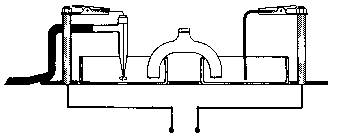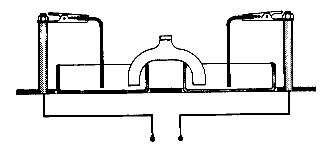## 20.4 Galvanic or Voltaic Cells

Chemical Concepts Demonstrated:  Voltaic/galvanic cells, relative half-cell potentials

Demonstration:• One of the dishes is filled with ZnSO4 and the other with HCl.
• A strip of Zn metal is attached at one end to the posts of the electrochemistry template and is placed at the other end into the dish filled with Zn2+.
• The hydrogen electrode is attached, placed into the HCl solution, and H2 gas is bubbled in. Insert the salt bridge.
• The Zn2+/Zn half-cell is replaced with a Cu2+/Cu half-cell.
• The H+/H2 half-cell is replaced with a Zn2+/Zn. (picture 2)Observations:

The potential in the absence of the salt bridge is 0.00 V.  After the salt bridge is inserted, the potential of
the first set up is around + 0.76 V and the cell is a galvanic or voltaic cell.  The Zn2+/Zn half-cell is the anode the H+/H2 is the cathode.

In the second set up, both the magnitude and the sign of the potential change.   The potential is now roughly - 0.34 V.

Picture 2 shows the third set up.  The potential is now - 1.10 V. If the leads are changed, the cell potential becomes + 1.10 V and the cell becomes a galvanic or voltaic cell.

Explanations (including important chemical equations):

With the leads connected so as to produce a cell potential of + 0.76 V, the half reactions are:

 anode: Zn (s) ---> Zn 2+ (aq) + 2 e- Eo = 0.76 V cathode: 2 H + (aq) + 2 e - ---> H2 (g) Eo = 0.00 V Zn (s) + 2 H +  (aq) ---> Zn 2+ (aq) + H2 (g) Eo cell = 0.76 V

If the standard-state potential for the H+/H2 half-cell is assumed to be 0.00 V, and the potential for the anode half-reaction is equal in magnitude but opposite in sign to the standard-state potential for the Zn2+/Zn couple, then the standard-state reduction potential for the Zn2+/Zn half-cell must be - 0.76 V.

If the Zn2+/Zn half-cell is replaced with a Cu2+/Cu half-cell without reversing the leads to the voltmeter, the overall cell potential is - 0.34 V and the standard-state reduction potential for the Cu2+/Cu couple is therefore + 0.34 V.

 anode: Cu (s) ---> Cu 2+ (aq) + 2 e- Eo =  - 0.34 V cathode: 2 H + (aq) + 2 e - ---> H2 (g) Eo =  - 0.00 V Cu (s) + 2 H +  (aq) ---> Cu 2+ (aq) + H2 (g) Eo cell  = -0.34 V

If the H+/H2 half-cell is replaced with a Zn2+/Zn half-cell, the overall cell potential should be - 1.10V.

 anode: Cu (s) ---> Cu 2+ (aq) + 2 e- Eo =  - 0.34 V cathode: Zn 2+ (aq) + 2 e- ---> Zn (s) Eo =  - 0.76 V Cu (s) + Zn 2+ (aq) ---> Cu 2+ (aq) + Zn (s) Eo cell  = -1.10 V

To set up a voltaic cell using these half reactions, one would have to reverse the leads to the voltmeter.